# NCERT Solutions for Class 9th: Ch 11 Constructions Maths

#### NCERT Solutions for Class 9th: Ch 11 Constructions Maths

Page No: 191

Exercise 11.1

1. Construct an angle of 90° at the initial point of a given ray and justify the construction.Steps of construction:

Step 1: A ray YZ is drawn.
Step 2: With Y as a centre and any radius, an arc ABC is drawn cutting YZ at C.
Step 3: With C as a centre and the same radius, mark a point B on the arc ABC.
Step 4: With B as a centre and the same radius, mark a point A on the arc ABC.
Step 5: With A and B as centre, draw two arcs intersecting each other with the same radius at X.
Step 6: X and Y are joined and a ray XY making an angle 90° with YZ is formed.

Justification for construction:
We constructed ∠BYZ = 60° and also ∠AYB = 60°.
Thus, ∠AYZ = 120°.
Also, bisector of ∠AYB is constructed such that:
∠AYB = ∠XYA ∠XYB
⇒ ∠XYB = 1/2∠AYB
⇒ ∠XYB = 1/2×60°
⇒ ∠XYB = 30°
Now,
∠XYZ = ∠BYZ + ∠XYB = 60° + 30° = 90°

2. Construct an angle of 45° at the initial point of a given ray and justify the construction.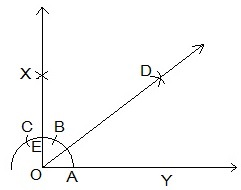Steps of construction:

Step 1: A ray OY is drawn.
Step 2: With O as a centre and any radius, an arc ABC is drawn cutting OY at A.
Step 3: With A as a centre and the same radius, mark a point B on the arc ABC.
Step 4: With B as a centre and the same radius, mark a point C on the arc ABC.
Step 5: With A and B as centre, draw two arcs intersecting each other with the same radius at X.
Step 6: X and Y are joined and a ray making an angle 90° with YZ is formed.
Step 7: With A and E as centres, two arcs are marked intersecting each other at D and the bisector of ∠XOY is drawn.

Justification for construction:
By construction,
∠XOY = 90°
We constructed the bisector of ∠XOY as DOY.
Thus,
∠DOY = 1/2 ∠XOY
∠DOY = 1/2×90° = 45°

3. Construct the angles of the following measurements:
(i) 30°        (ii) 22.5°        (iii) 15°

(i) 30°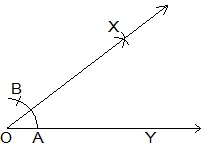Steps of constructions:
Step 1: A ray OY is drawn.
Step 2: With O as a centre and any radius, an arc AB is drawn cutting OY at A.
Step 3: With A and B as centres, two arcs are marked intersecting each other at X and the bisector of is drawn.
Thus, ∠XOY is the required angle making 30° with OY.

(ii) 22.5°Steps of constructions:
Step 1: An angle ∠XOY = 90° is drawn.
Step 2: Bisector of ∠XOY is drawn such that ∠BOY = 45° is constructed.
Step 3: Again, ∠BOY is bisected such that ∠AOY is formed.
Thus, ∠AOY is the required angle making 22.5° with OY.

(iii) 15°Steps of constructions:
Step 1: An angle ∠AOY = 60° is drawn.
Step 2: Bisector of ∠AOY is drawn such that ∠BOY = 30° is constructed.
Step 3: With C and D as centres, two arcs are marked intersecting each other at X and the bisector of ∠BOY is drawn.
Thus, ∠XOY is the required angle making 15° with OY.

4. Construct the following angles and verify by measuring them by a protractor:
(i) 75°         (ii) 105°        (iii) 135°

(i) 75°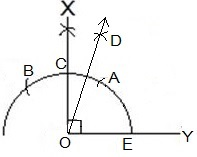Steps of constructions:
Step 1: A ray OY is drawn.
Step 2: An arc BAE is drawn with O as a centre.
Step 3: With E as a centre, two arcs are A and C are made on the arc BAE.
Step 4: With A and B as centres, arcs are made to intersect at X and ∠XOY = 90° is made.
Step 5: With A and C as centres, arcs are made to intersect at D
Step 6: OD is joined and and ∠DOY = 75° is constructed.
Thus, ∠DOY is the required angle making 75° with OY.

(ii) 105°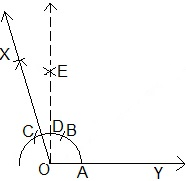Steps of constructions:
Step 1: A ray OY is drawn.
Step 2: An arc ABC is drawn with O as a centre.
Step 3: With A as a centre, two arcs are B and C are made on the arc ABC.
Step 4: With B and C as centres, arcs are made to intersect at E and ∠EOY = 90° is made.
Step 5: With B and C as centres, arcs are made to intersect at X
Step 6: OX is joined and and ∠XOY = 105° is constructed.
Thus, ∠XOY is the required angle making 105° with OY.

(iii) 135°Steps of constructions:Step 1: A ray DY is drawn.
Step 2: An arc ACD is drawn with O as a centre.
Step 3: With A as a centre, two arcs are B and C are made on the arc ACD.
Step 4: With B and C as centres, arcs are made to intersect at E and ∠EOY = 90° is made.
Step 5: With F and D as centres, arcs are made to intersect at X or bisector of ∠EOD is constructed.
Step 6: OX is joined and and ∠XOY = 135° is constructed.
Thus, ∠XOY is the required angle making 135° with DY.

5. Construct an equilateral triangle, given its side and justify the construction.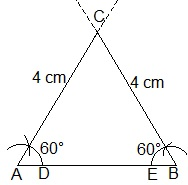Steps of constructions:
Step 1: A line segment AB=4 cm is drawn.
Step 2: With A and B as centres, two arcs are made.
Step 4: With D and E as centres, arcs are made to cut the previous arc respectively and forming angle of 60° each.
Step 5: Lines from A and B are extended to meet each other at C.
Thus, ABC is the required triangle formed.

Justification:
By construction,
AB = 4 cm, ∠A = 60° and ∠B = 60°
We know that,
∠A ∠B ∠C = 180° (Sum of the angles of a triangle)
⇒ 60° + 60° + ∠C = 180°
⇒ 120° + ∠C = 180°
⇒ ∠C = 60°
BC = CA = 4 cm (Sides opposite to equal angles are equal)
AB = BC = CA = 4 cm
∠A = ∠B = ∠C = 60°

Page No: 195

Exercise 11.2

1. Construct a triangle ABC in which BC = 7cm, ∠B = 75° and AB + AC = 13 cm.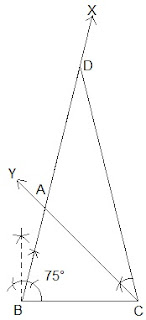Steps of Construction:
Step 1: A line segment BC of 7 cm is drawn.
Step 2: At point B, an angle ∠XBC is constructed such that it is equal to 75°.
Step 3: A line segment BD = 13 cm is cut on BX (which is equal to AB+AC).
Step 3: DC is joined and ∠DCY = ∠BDC is made.
Step 4: Let CY intersect BX at A.
Thus, ΔABC is the required triangle.

2. Construct a triangle ABC in which BC = 8cm, ∠B = 45° and AB - AC = 3.5 cm.Steps of Construction:
Step 1: A line segment BC = 8 cm is drawn and at point B, make an angle of 45° i.e. ∠XBC.
Step 2: Cut the line segment BD = 3.5 cm (equal to AB - AC) on ray BX.
Step 3: Join DC and draw the perpendicular bisector PQ of DC.
Step 4: Let it intersect BX at point A. Join AC.
Thus, ΔABC is the required triangle.

3. Construct a triangle PQR in which QR = 6cm, ∠Q = 60° and PR – PQ = 2cm.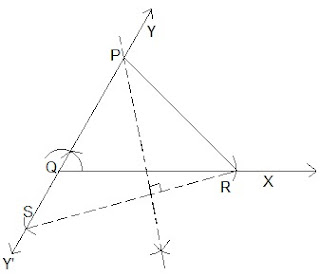Steps of Construction:
Step 1: A ray QX is drawn and cut off a line segment QR = 6 cm from it.
Step 2:. A ray QY is constructed making an angle of 60º with QR and YQ is produced to form a line YQY'
Step 3: Cut off  a line segment QS = 2cm  from QY'. RS is joined.
Step 5: Draw perpendicular bisector  of RS intersecting QY at a point  P. PR is joined.
Thus, ΔPQR is the required triangle.

4. Construct a triangle XYZ in which ∠Y = 30°, ∠Z = 90° and XY + YZ + ZX = 11 cm.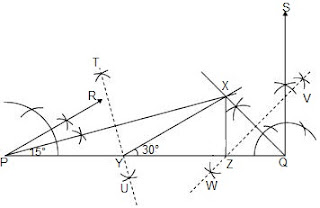Steps of Construction:
Step 1: A line segment PQ = 11 cm is drawn. (XY + YZ + ZX = 11 cm)
Step 2: An angle, ∠RPQ = 30° is constructed at point A and an angle ∠SQP = 90° at point B.
Step 3: ∠RPQ and ∠SQP are bisected . The bisectors of these angles intersect each other at point X.
Step 4: Perpendicular bisectors TU of PX and WV of QX are constructed.
Step V: Let TU intersect PQ at Y and WV intersect PQ at Z. XY and XZ are joined.
Thus, ΔXYZ is the required triangle.

5. Construct a right triangle whose base is 12cm and sum of its hypotenuse and other side is 18 cm.